# Working with .m Files in LabVIEW for Text-Based Signal Processing, Analysis, and Math

Publish Date: Jun 07, 2018 | 11 Ratings | 3.45 out of 5 | Print | 1 Customer Review | Submit your review

## Overview

This tutorial provides several step-by-step exercises that introduce you to using NI LabVIEW software to work with .m file scripts for text-based math, signal processing, and analysis. This tutorial also explores LabVIEW MathScript, the LabVIEW feature that offers this capability.

Note: LabVIEW MathScript is available in the Full and Professional Development Systems in LabVIEW 8.0 through 8.6. In LabVIEW 2009, LabVIEW MathScript becomes the LabVIEW MathScript RT Module. You cannot run VIs from previous versions of LabVIEW that contain MathScript Nodes until you install and activate the MathScript RT Module.

This tutorial does not provide an extensive introduction to the LabVIEW development environment. Refer to the Getting Started with LabVIEW Virtual Instruments tutorial (linked below) for a general overview of LabVIEW.

### 1. Working with .m Files in LabVIEW

Although LabVIEW is a development environment built around a graphical programming language, LabVIEW also allows you to create .m files and work with text-based math. One way to work with text-based math in LabVIEW is through a script node interface. Script nodes combine text-based .m files with traditional LabVIEW graphical programming. Script nodes are resizable text-entry regions you can add to LabVIEW block diagrams.

One type of script node, the MATLAB script node, calls the MATLAB® software to execute scripts. You must have a licensed copy of the MATLAB software installed on your computer to use MATLAB script nodes because the script nodes invoke the MATLAB software script server to execute scripts written in the MATLAB language syntax.

You also can create scripts in LabVIEW with LabVIEW MathScript. LabVIEW MathScript is a text-based language you can use to write functions and scripts for use in the LabVIEW MathScript Window or MathScript Node. MathScript Nodes can process many of your text-based scripts created in a MATLAB or compatible environment. However, because the MathScript RT Module engine does not support all functions supported by the MATLAB software, some functions in your existing scripts might not be supported. You can implement such functions with a Formula Node or another script node. You can use the LabVIEW Application Builder and MathScript to build stand-alone applications and shared libraries that include functionality defined in .m files.

You can interact with MathScript in several different ways. Use the LabVIEW MathScript Window for an interactive interface in which you can load, save, develop, and execute .m file scripts. Use the MathScript Node to utilize the graphical programming environment in LabVIEW and to deploy .m file scripts as part of a stand-alone application.

The following section offers a brief walk-through of the LabVIEW MathScript Window. Later sections in this tutorial discuss the MathScript Node.

MATLAB® is a registered trademark of The MathWorks, Inc. All other trademarks are the property of their respective owners

### 2. Working in the LabVIEW MathScript Window

The Getting Started window appears when you launch LabVIEW. Use this window to create new VIs and projects, select among the most recently opened LabVIEW files, find examples, and launch the LabVIEW Help. You also can access information and resources to help you learn about LabVIEW, such as specific manuals, help topics, and resources on the National Instruments Web site.

### Opening the LabVIEW MathScript Window

1. Select Tools»MathScript Window to display the LabVIEW MathScript Window.
1. The LabVIEW MathScript Window includes a Command Window in the lower left corner. Enter the following command in the Command Window and press the <Enter> key:

t = 1:10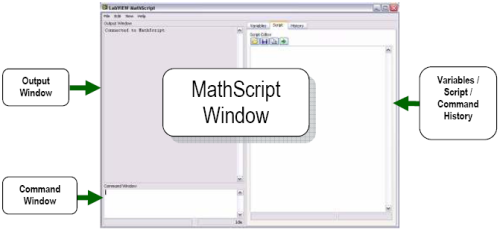1. Notice that the result of the command you entered appears in the Output Window, located just above the Command Window. The result of this command is a vector of ten elements, where the elements start at 1 and end at 10 with a step size of 1.
2. Locate the tabs labeled Variables, Script, and History at the top right of the LabVIEW MathScript Window. Click the Variables tab to display the Variables page. This page displays a list of all variables you define and previews variables that you select.
3. Notice that the variable list contains an entry for the t vector you defined in step 2. Click the t in the variable list to display the contents of t in the Preview Pane located in the lower right corner of the LabVIEW MathScript Window.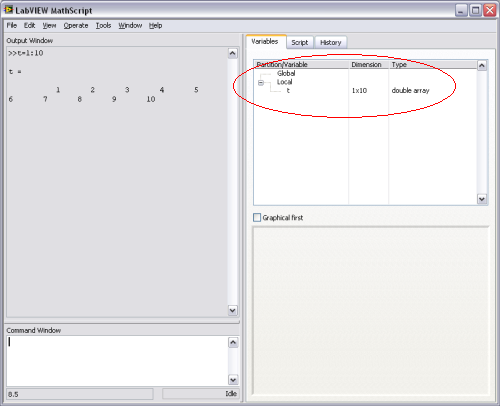1. Enter the following commands in the Command Window. Press the <Enter> key at the end of each line.

t=0:.1:2*pi;
y=sin(t);

After you press the <Enter> key, LabVIEW displays each command in the Output Window. LabVIEW does not display the output for each command because the semicolon at the end of each line directs LabVIEW to suppresses the output.
2. On the Variables page, click the local variable y. Then modify the view options on the Variables page to view the contents of the y variable as a graph or as numerical elements, as shown in the following figure. You also can use these different views to modify the contents of the variable.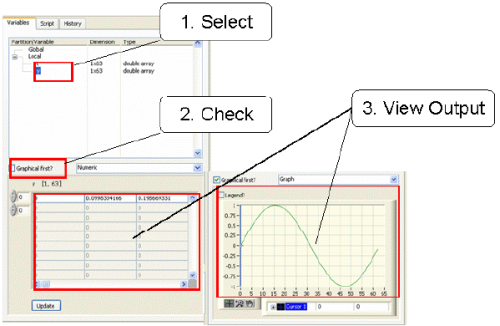#### Working with Multi-Line Scripts

The previous exercise demonstrated how to use the Command Window to enter commands and view the output for these commands. The exercise also showed how to view and modify the contents of variables. The following exercise shows how to use the Script Editor to enter multi-line scripts and create larger, more involved scripts. Complete the following steps to create a multi-line script, run the script, and then display the results in several different display formats.

1. Click the Script tab to display the Script page.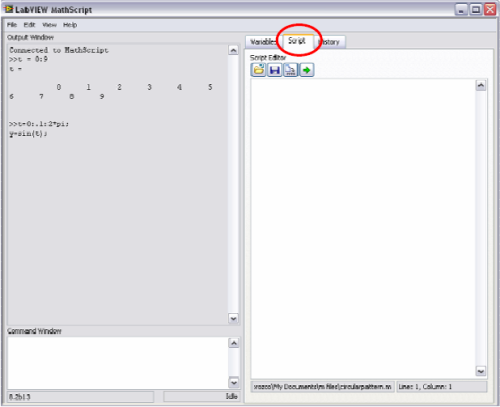1. Copy and paste the following file script into the Script Editor on the Script page. Notice the script includes percent signs (%) that precede lines of comments. Use these comments to include help documentation for the script.

% This example calculates and graphs the theoretical
% BER plots for two types of communication
% systems (BPSK and QPSK)
Eb_over_No_in_dB = [0:14];
% Purposes of the x-axis of the plot
Eb_No=10.^(Eb_over_No_in_dB./10);
% PSK is antipodal
xant = sqrt(2.*Eb_No);
% QPSK is orthogonal
xorth = sqrt(Eb_No);
% Use the erfc function as equivalent to
% Q function
Qant = 0.5*erfc(xant/sqrt(2));
Qorth = 0.5*erfc(xorth/sqrt(2));
% Plot the first result
semilogy(Eb_over_No_in_dB,Qant);
% Keep the same plot in graph
% plot the second one
grid on; hold on;
semilogy(Eb_over_No_in_dB,Qorth,'r--');
v = axis;
axis([v(1:2) 10^-6 .1])
xlabel('Eb/N0 in dB');
ylabel('Probability of bit error')
hold off;
legend('Antipodal','Orthogonal')

2. Click the Run button to execute the script, as shown in the following figure.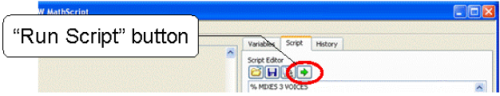The example script plots the theoretical value of bit error rate (BER) from a digital communication system with two different digital modulation schemes.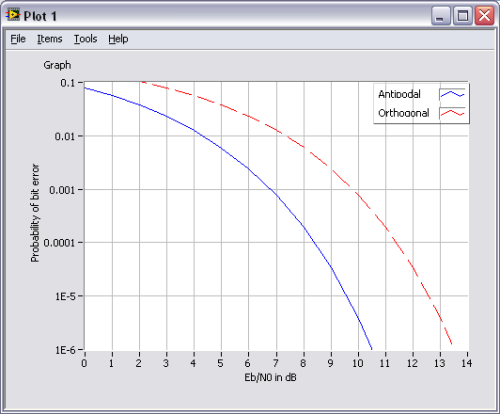This example invokes several built-in functions for math, graph formatting, and other tasks. MathScript includes built-in functions for a variety of tasks, including categories such as advanced math, approximation, audio, basic math, bitwise operations, Boolean operations, utility commands, relational operators, data acquisition, digital signal processing, digital filter design, digital filter implementation, combinatorial geometry, interfacing with shared libraries (DLLs), linear algebra, linear systems, matrix operations, set membership, modeling/prediction, ODE, optimization, plotting, polynomial operations, programming constructs, resampling functions, set operations, string manipulation, support functions, time/date functions, timing functions, transforms, trigonometric functions, vector analysis, waveform generation, and window generation.

In addition to the built-in MathScript functions, you also can define functions in .m files. Complete the following steps to define a function in a .m file.

1. Delete all commands on the Script Editor page. Then copy and paste the following script into the Script Editor. The script defines a function named rad2deg. This function converts an input parameter rad to degrees and returns the result in the deg output.

% This is a Comment
% This function converts from radians to degrees.

1. Click the Save button to save the function as rad2deg.m in the LabVIEW Data directory. By default, LabVIEW searches the LabVIEW Data  directory for user-defined functions and user-defined scripts.

NOTE: Use the MathScript: Search Paths Options page to configure the default search path list for MathScript. Select File»LabVIEW MathScript Properties to display the LabVIEW MathScript Properties dialog box and select MathScript: Search Paths from the Category list to display this page.
2. In the Command Window, enter the following commands and press the <Enter> key after each command.
 Command Result of the Command rad2deg(pi) Invokes the user-defined function you previously defined. help rad2deg Returns the first commented paragraph as the help documentation for the user-defined function. rad2deg(linspace(0,pi,10)) Invokes the user-defined function that you previously defined with a function call (“linspace”) as a parameter.

The user-defined function rad2deg now is available as a function you can call from scripts.
Refer to the MathScript Function Syntax topic and the Calling User-Defined Functions from LabVIEW MathScript topic in the LabVIEW Help (linked below) for more information about working with user-defined functions.

### 3. MathScript Tips

This section contains a few tips for using the LabVIEW MathScript Window. The following tips might be useful as you work in the LabVIEW MathScript Window.

• Place the cursor in the Command Window and use the Up and Down arrow keys on the keyboard to scroll through the command history.
• Right click the Preview Pane on the Variables page and select Undock Window from the shortcut menu to display the variable in a separate window that you can resize.

### 4. Trying Out .m File Scripts

There are several online and local options that allow you to test your user-defined scripts in MathScript. Refer to the Use Fully Featured LabVIEW MathScript Today Developer Zone document (linked below) for more information about these options.

### 5. Using the MathScript Node, Part I

Have you ever wanted to interactively change the value of a parameter and immediately see the response? Have you ever wanted to test the algorithm in a user-defined script with real acquired data?

With LabVIEW, you can choose the most effective syntax for technical computing whether you are developing algorithms, exploring signal processing concepts, or analyzing results. You can combine LabVIEW graphical programming with LabVIEW MathScript.

You can use the MathScript Node to combine textual algorithms with LabVIEW graphical programming. You then can use knobs, slides, buttons, graphics, and other controls and indicators to instrument .m file scripts.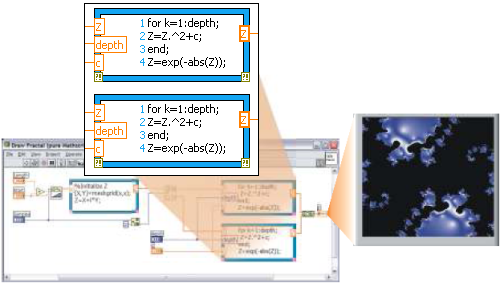### Creating the User Interface

This section describes how to build an example in LabVIEW that highlights the benefits of combining text-based MathScripts with LabVIEW graphical programming.

Complete the following steps to create controls and indicators in a LabVIEW front panel window.

1. In the Getting Started window, select File»New VI or select Blank VI from the New section to create a new VI.
1. Add a Waveform Graph indicator to the front panel. Double-click the Waveform Graph text label to edit the label. Change the name of the label to Signal Samples.
2. Add a second Waveform Graph indicator to the front panel. Change the label to FFT Result.
3. Switch to the block diagram and place a MathScript Node on the block diagram.

NOTE: You can enter commands in the MathScript Node in one of two ways. First, you can enter commands directly in the node. Second, you can import the script from an existing .m file.

1. Copy and paste the following script into the MathScript Node:

fftresult=abs(fft(signalin));
fftresult=fftresult(1:end/2);

You also can load and save scripts from .m files by right-clicking the border of the MathScript Node and selecting Import or Export from the shortcut menu.

The first command line in the previous script does the following:

• Calls the fft function to apply a fast Fourier transform (FFT) to an input vector called signalin.
• Calls the abs function to calculate the absolute value of the result of the fft function.
• Assigns the result to a variable called fftresult.

The second command line in the previous script assigns the fftresult variable to the first half of the data that is the result of the analysis of the previous line. The reason for this step is that the result of applying an FFT to a real-valued signal is symmetric, so it is common to examine only half of the result.

1. Right-click the left side of the MathScript Node frame and select Add Input from the shortcut menu. Enter signalin in the input terminal to add an input for the signalin variable in the script.
2. Right-click the right side of the MathScript Node frame and select Add Output from the shortcut menu. Enter fftresult in the output terminal to add an output for the fftresult variable in the script.
3. Right-click the fftresult output terminal and select Choose Data Type»1D-Array»DBL 1D from the shortcut menu to specify the data type of the fftresult output variable.
1. Add a While Loop to the block diagram so that the loop encloses all the elements on the block diagram, as shown in the following block diagram. The While Loop allows the acquisition and analysis in the code to run continuously.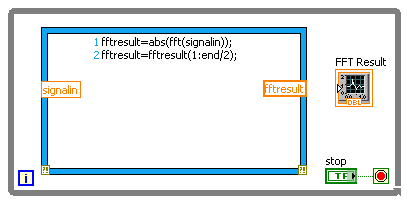1. Right-click the conditional terminal of the While Loop and select Create Control to add a Stop button to the front panel.
2. Add the Simulate Signal Express VI to the block diagram, inside the While Loop to the left of the MathScript Node. The Simulate Signal Express VI simulates acquiring data from a measurement device.
3. Choose the following configuration options in the Simulate Signal dialog box and then click the OK button.

Signal

• Signal type = Square
• Frequency = 50 Hz
• Noise type = Uniform White Noise
• Noise amplitude = 0.3

Timing

• Samples per second (Hz) = 10000
• Automatic = checked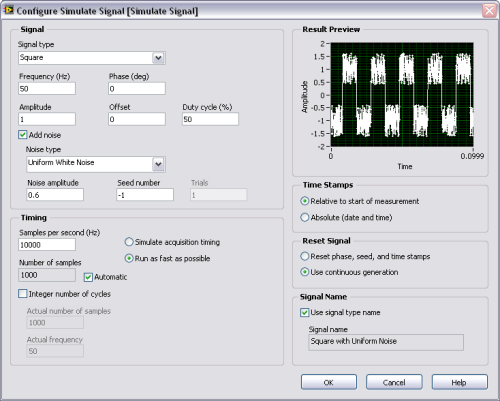1. Connect the data output from the Simulate Signal Express VI to the Signal Samples graph to display the synthesized signal.
2. Add a Convert from Dynamic Data Express VI to the block diagram. Click the  OK button to choose the default settings for the VI and close the configuration dialog box.

The Convert from Dynamic Data Express VI converts data from the dynamic data type to a 1D array of scalars, which is a data type that the MathScript Node supports.

1. Wire the output from the Simulate Signal Express VI to the input of the Convert from Dynamic Data Express VI.
2. Wire the output of the Convert from Dynamic Data VI to the signalin input of the MathScript Node.
3. Wire the fftresult output on the right side of the MathScript Node to the FFT Result graph terminal. The block diagram should resemble the following screenshot.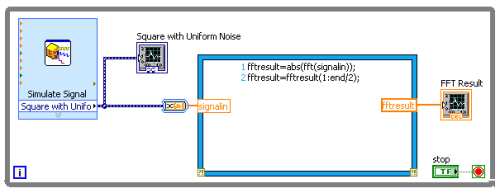1. Switch to the front panel and click the Run button to run the VI. LabVIEW displays the live signal in the graph on the left side of the front panel and the results of the analysis in the graph on the right side of the front panel.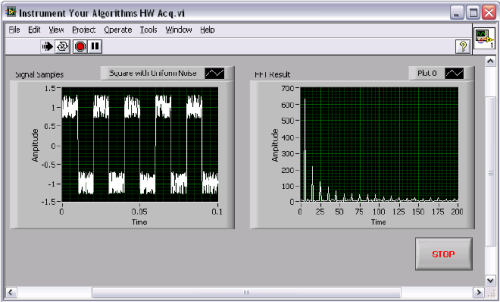1. Click the STOP button and then switch to the block diagram.
2. Add a Filter Express VI to the block diagram inside the While Loop.
3. Choose the following selections in the Filter dialog box and click the OK button.
• Filter type = smoothing
• Moving average = Triangular
• Half-width moving average = 7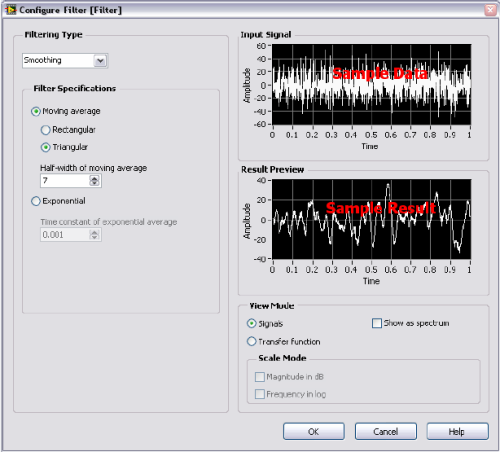1. Wire the output of the Simulate Signal Express VI to the Signal input of the Filter VI.
2. Right click the Filtered Signal output of the Filter VI and select Create»Graph Indicator from the shortcut menu to create a waveform graph. This graph displays the filtered signal. The block diagram should resemble the following screenshot.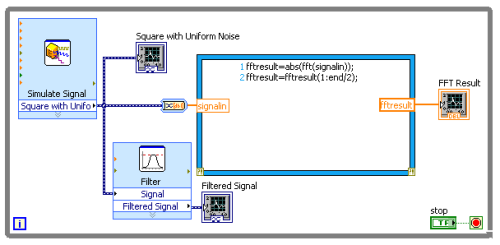1. Switch to the front panel and click the Run button to run the VI.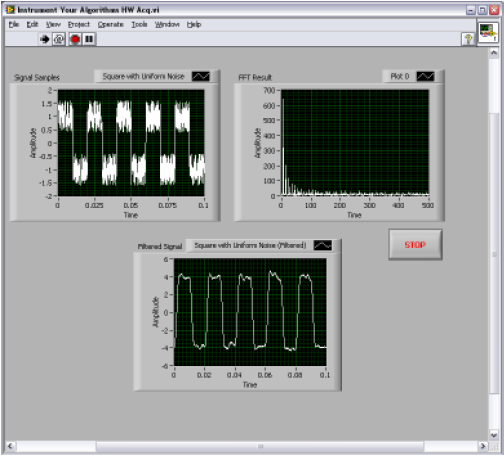### 6. Using the MathScript Node, Part II

This section includes an example of building a more complicated VI that displays an RF antenna pattern for a dish antenna in linear (XY) and polar plots. The example shows how to build a custom user interface to add interactivity to an algorithm you define in a script in a .m file using a MathScript Node. The example adds a control input to set the amplitude/lambda input parameter of the algorithm.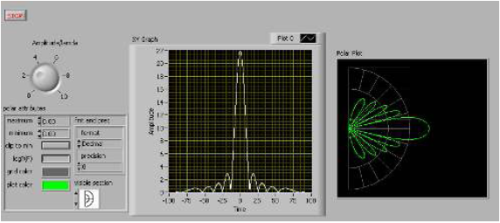### Creating the User Interface

1. Open a new VI.
2. Add a knob control to the front panel. You can use this control to set the size and wavelength of the antenna.
1. Change the name of the Knob control to Amplitude/lambda.
2. Add an XY graph to the front panel. You can display the results of an application by using indicators, charts, and other graphical displays on the front panel of a VI.
1. Add a polar plot indicator to the front panel. You can use this indicator to show the RF antenna pattern as a function of the angle.
1. Switch to the block diagram and locate the controls and indicators you created on the front panel. Move the polar plot indicator and the XY graph to the right side of the block diagram, as shown in the following screenshot.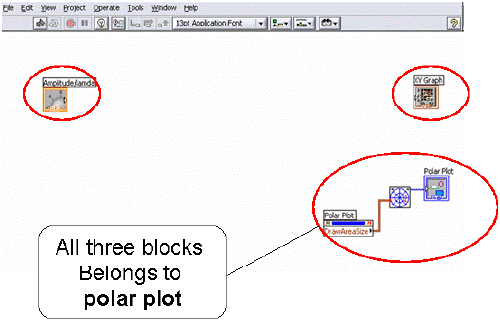### Adding a Script to a MathScript Node

1. Add a MathScript Node to the block diagram.
2. Copy and paste the following script into the MathScript Node. This script processes the gain of the antenna at different angles.

% Create angle vector in radians
theta = linspace(-pi/2,pi/2,1000);

u = 2*pi*a*sin(theta);

% initialize matrix
E = ones(size(u));

% Get index of non-zero values
i = find(u);

% Evaluate Antenna pattern equation
E(i) = pi*a^2*abs(2*besselj(1,u(i))./(u(i)));

% change theta to degrees units for polar plot
Out=theta.*180./pi;

3. Right-click the left border of the MathScript Node and select Add Input from the shortcut menu.
4. Enter a in the input to add an input for the a variable in the script.
5. Right-click the right border of the MathScript Node and select Add Output from the shortcut menu.
6. Enter Out in the output terminal to add an output for the Out variable in the script. This variable represents the angle vector for plotting purposes.
7. Right-click the Out output terminal and select Choose Data Type»1D-Array»DBL 1D from the shortcut menu to specify the data type of the Out output variable.
8. Repeat the steps 5 through 7 for the E variable. This variable represents the gain output at different angles.
9. Add a Bundle function to the block diagram to combine the X and Y components of the regular plot, as shown in the following block diagram.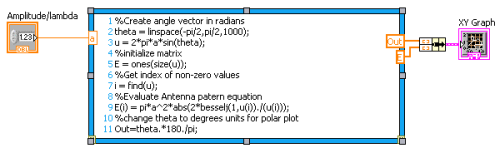1. Add a For Loop to the block diagram.
2. Place a Bundle function inside the For Loop.
3. Connect the input and output terminals. The squares in the border of the For Loop indicate the loop is set to auto index, or process each input element separately.
4. Right click the Polar attributes input of the Polar Plot VI and select Create»Control from the shortcut menu.
5. Switch to the front panel and set the Amplitude/lambda control to 2. Configure the parameters of the polar plot to show a Log scale and display only the right half of the plot, as shown in the following front panel.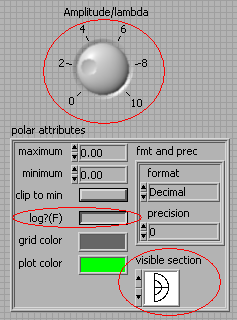1. Run the VI. The graph updates and then the VI automatically stops.
2. Switch to the block diagram. Add an express While Loop that encircles all elements on the block diagram, as shown in the following screenshot.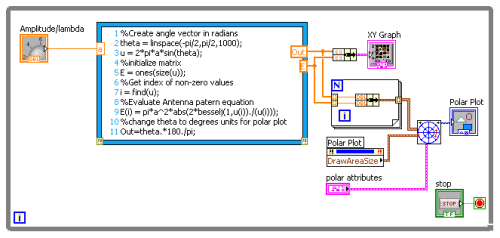1. Run the VI. The results represent the gain of a dish antenna at different angles.LabVIEW MathScript Homepage

LabVIEW 2018 Help: LabVIEW MathScript Window

LabVIEW 2018 Help: MathScript Node

Developer Zone: Use Fully Featured LabVIEW and MathScript Today

Customer Reviews
1 Review | Submit your review

- Apr 25, 2007